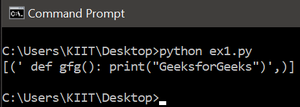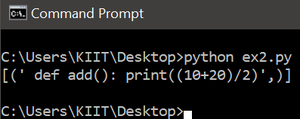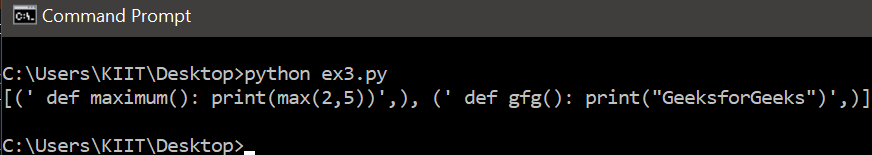# How to store Python functions in a Sqlite table?

• Last Updated : 01 Oct, 2020

SQLite is a relational database system contained in a C library that works over syntax very much similar to SQL. It can be fused with Python with the help of the sqlite3 module. The Python Standard Library sqlite3 was developed by Gerhard Häring. It delivers an SQL interface compliant with the DB-API 2.0 specification described by PEP 249.

To use this module and establish the connection with database:-

Attention geek! Strengthen your foundations with the Python Programming Foundation Course and learn the basics.

To begin with, your interview preparations Enhance your Data Structures concepts with the Python DS Course. And to begin with your Machine Learning Journey, join the Machine Learning - Basic Level Course

1. Import sqlite3 module and create the object representing the database.

```import sqlite3
conn = sqlite3.connect('function.db')
```

2. To execute SQL commands, construct a cursor object and call the execute() method.

```c = conn.cursor()
c.execute('''CREATE TABLE student
(Number REAL,stud_name TEXT)''')
```

Note: In Order to track the operations on a database level we need to download the SQLite browser database.

Steps to store Python function in SQLite table:

• Step 1) Import sqlite3 and create the object of database .

## Python3

 `import` `sqlite3``conn ``=` `sqlite3.connect(``'function.db'``)`

Here we have imported sqlite3 module, and We have initialized the database function.db. This instruction will create the database if the database doesn’t exist and if the database having the same name as defined exist than it will move further.

• Step 2) Create a Cursor object and call its execute() method to create table.

## Python3

 `c ``=` `conn.cursor()``c.execute(``'''CREATE TABLE pyfuction``             ``(func_defination TEXT)'''``)`

After initializing the database we have to create the cursor object using conn.cursor(). The cursor allows us to execute the SQL commands.

• Step 3) Write Function in below syntax.

## Python3

 `code ``=` `""" def gfg(): print("GeeksforGeeks") """`

Since we have to store the function definition inside the table, we have to write function in the above syntax.

• Step 4) Insert function into pyfunction table.

## Python3

 `c.execute(``"INSERT INTO pyfuction (func_defination) VALUES (?)"``,``          ``(code,))``conn.commit()`

The SQL command “INSERT INTO pyfuction (func_defination) VALUES (?)”, (code) inserts the value of variable code inside the table pyfunction in the form of text. Then use the commit() method to save the changes.

Below are some examples store Python functions in a Sqlite table?

Example 1: Storing gfg() function in sqlite table named pyfunction.

## Python3

 `# Importing required library``import` `sqlite3`` ` `# Initializing the database``conn ``=` `sqlite3.connect(``'function.db'``)`` ` `# Creating cursor object``c ``=` `conn.cursor()`` ` `# Creating table``c.execute(``'''CREATE TABLE pyfuction``             ``(func_defination TEXT)'''``)`` ` `# Storing a python function in the Sqlite table``code ``=` `""" def gfg(): print("GeeksforGeeks")"""``c.execute(``"INSERT INTO pyfuction (func_defination) VALUES (?)"``,``          ``(code))`` ` `# Terminating the transaction``conn.commit()`

Output:Example 2: Here is another example of storing a function named add() in the pyfunction table.

## Python3

 `# Importing required library``import` `sqlite3`` ` `# Initializing the database``conn ``=` `sqlite3.connect(``'function.db'``)`` ` `# Creating cursor object``c ``=` `conn.cursor()`` ` `# Creating table``c.execute(``'''CREATE TABLE pyfuction``             ``(func_defination TEXT)'''``)`` ` `# Storing a python function in the Sqlite table``code ``=` `""" def add(): print((10+20)/2)"""``c.execute(``"INSERT INTO pyfuction (func_defination) VALUES (?)"``,``          ``(code,))`` ` `# Displaying Content of the table``c.execute(``"SELECT * FROM pyfuction;"``)``print``(c.fetchall())`` ` `# Terminating the transaction``conn.commit()`

Output:Example 3: Storing two functions, maximum() and gfg() in pyfunction table.

## Python3

 `# Importing required library``import` `sqlite3`` ` `# Initializing the database``conn ``=` `sqlite3.connect(``'function.db'``)`` ` `# Creating cursor object``c ``=` `conn.cursor()`` ` `# Creating table``c.execute(``'''CREATE TABLE pyfuction``             ``(func_defination TEXT)'''``)`` ` `# Storing a python function in the Sqlite table``def1 ``=` `""" def maximum(): print(max(2,5))"""``c.execute(``"INSERT INTO pyfuction (func_defination) VALUES (?)"``,``          ``(def1,))`` ` `# Storing another python function in the Sqlite table``def2 ``=` `""" def gfg(): print("GeeksforGeeks")"""``c.execute(``"INSERT INTO pyfuction (func_defination) VALUES (?)"``,``          ``(def2,))`` ` `# Displaying Content of the table``c.execute(``"SELECT * FROM pyfuction;"``)``print``(c.fetchall())`` ` `# Terminating the transaction``conn.commit()`

Output:My Personal Notes arrow_drop_up